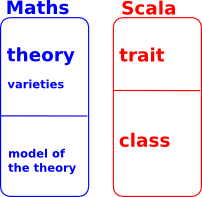# Computer Algebra Program using Scala

For a long time I have wanted to program a computer algebra system (CAS) using the Scala programming language. However I have never really found a convincing design that would do what I want (A few years ago I previously asked this type of question on forums). Perhaps now the capabilities of Scala have increased, perhaps also my own knowledge has increased, although I am far from being an expert on any of this stuff. Perhaps now might be the time to ask again?

By 'computer algebra system (CAS)' I mean a program that will handle, not just numerical mathematics, but also symbolic algebra, discrete algebra, mathematical logic and so on.

So how do we specify and design such a program? Is there some way to break mathematics down into manageable parts? From what little I know about Gödel's theorems I think we can't derive a canonical way to do this but mathematicians, over the years, have evolved ways to break down the subject.

## Universal Algebra

One approach is 'universal algebra'. We can divide the subject into:

• Theories
• Varieties of those theories
• Models of those theories.

So theories are things like:

• Set theory
• Graph Theory
• Group Theory
• .. and so on.

This links up quite nicely with Scala in that:

• Theories could correspond to traits.
• Models could correspond to classes.
• Varieties could correspond to traits with type parameters
 However, they don't quite line up, in particular: We need dependant types in type constructors We there is no way to enforce axioms in traits.### Example Vectors

As an example to illustrate this lets look at 'vectors' (the mathematical concept of vector, not what the java class library uses the word for).

 ```trait Field { def *(a:Field,b:Field) def +(a:Field,b:Field) // other methods ommited for this example }   trait Vector2d[Scaler <: Field] { def *(a:Scaler,b:Field) def +(a:Field,b:Field) // other methods ommited for this example } ```